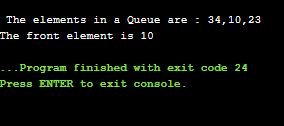# Circular Queue

### Why was the concept of the circular queue introduced?

There was one limitation in the array implementation of [Queue]. If the rear reaches to the end position of the Queue then there might be possibility that some vacant spaces are left in the beginning which cannot be utilized. So, to overcome such limitations, the concept of the circular queue was introduced.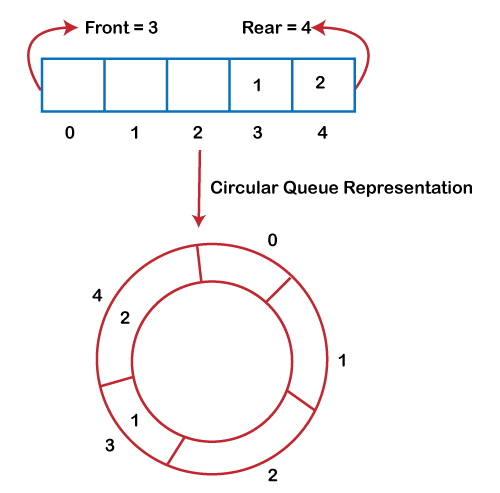As we can see in the above image, the rear is at the last position of the Queue and front is pointing somewhere rather than the 0th position. In the above array, there are only two elements and other three positions are empty. The rear is at the last position of the Queue; if we try to insert the element then it will show that there are no empty spaces in the Queue. There is one solution to avoid such wastage of memory space by shifting both the elements at the left and adjust the front and rear end accordingly. It is not a practically good approach because shifting all the elements will consume lots of time. The efficient approach to avoid the wastage of the memory is to use the circular queue data structure.

### What is a Circular Queue?

A circular queue is similar to a linear queue as it is also based on the FIFO (First In First Out) principle except that the last position is connected to the first position in a circular queue that forms a circle. It is also known as a Ring Buffer .

### Operations on Circular Queue

The following are the operations that can be performed on a circular queue:

• Front: It is used to get the front element from the Queue.
• Rear: It is used to get the rear element from the Queue.
• enQueue(value): This function is used to insert the new value in the Queue. The new element is always inserted from the rear end.
• deQueue(): This function deletes an element from the Queue. The deletion in a Queue always takes place from the front end.

### Applications of Circular Queue

The circular Queue can be used in the following scenarios:

• Memory management: The circular queue provides memory management. As we have already seen that in linear queue, the memory is not managed very efficiently. But in case of a circular queue, the memory is managed efficiently by placing the elements in a location which is unused.
• CPU Scheduling: The operating system also uses the circular queue to insert the processes and then execute them.
• Traffic system: In a computer-control traffic system, traffic light is one of the best examples of the circular queue. Each light of traffic light gets ON one by one after every jinterval of time. Like red light gets ON for one minute then yellow light for one minute and then green light. After green light, the red light gets ON.

### Enqueue operation

The steps of enqueue operation are given below:

• First, we will check whether the Queue is full or not.
• Initially the front and rear are set to -1. When we insert the first element in a Queue, front and rear both are set to 0.
• When we insert a new element, the rear gets incremented, i.e., rear=rear+1 .

### Scenarios for inserting an element

There are two scenarios in which queue is not full:

• If rear != max - 1 , then rear will be incremented to mod(maxsize) and the new value will be inserted at the rear end of the queue.
• If front != 0 and rear = max - 1 , it means that queue is not full, then set the value of rear to 0 and insert the new element there.

There are two cases in which the element cannot be inserted:

• When front ==0 && rear = max-1 , which means that front is at the first position of the Queue and rear is at the last position of the Queue.
• front== rear + 1;

Algorithm to insert an element in a circular queue

Step 1: IF (REAR+1)%MAX = FRONT
Write " OVERFLOW "
Goto step 4
[End OF IF]

Step 2: IF FRONT = -1 and REAR = -1
SET FRONT = REAR = 0
ELSE IF REAR = MAX - 1 and FRONT ! = 0
SET REAR = 0
ELSE
SET REAR = (REAR + 1) % MAX
[END OF IF]

Step 3: SET QUEUE[REAR] = VAL

Step 4: EXIT

### Dequeue Operation

The steps of dequeue operation are given below:

• First, we check whether the Queue is empty or not. If the queue is empty, we cannot perform the dequeue operation.
• When the element is deleted, the value of front gets decremented by 1.
• If there is only one element left which is to be deleted, then the front and rear are reset to -1.

Algorithm to delete an element from the circular queue

Step 1: IF FRONT = -1
Write " UNDERFLOW "
Goto Step 4
[END of IF]

Step 2: SET VAL = QUEUE[FRONT]

Step 3: IF FRONT = REAR
SET FRONT = REAR = -1
ELSE
IF FRONT = MAX -1
SET FRONT = 0
ELSE
SET FRONT = FRONT + 1
[END of IF]
[END OF IF]

Step 4: EXIT

Let’s understand the enqueue and dequeue operation through the diagrammatic representation.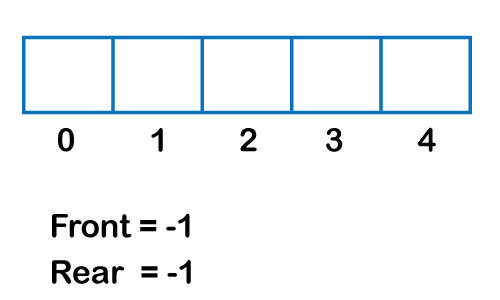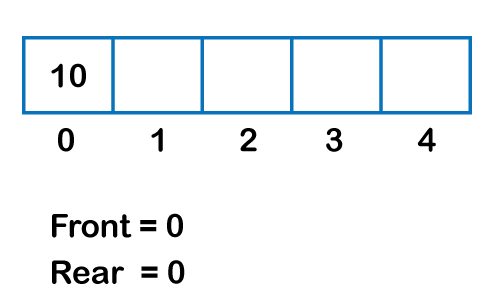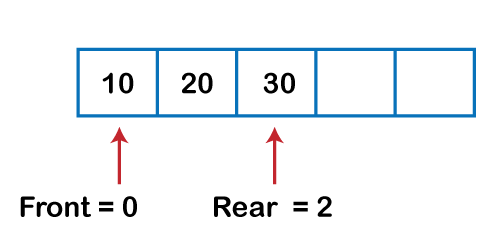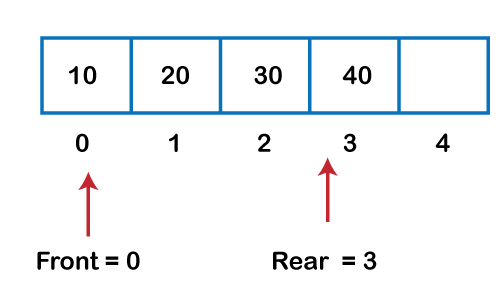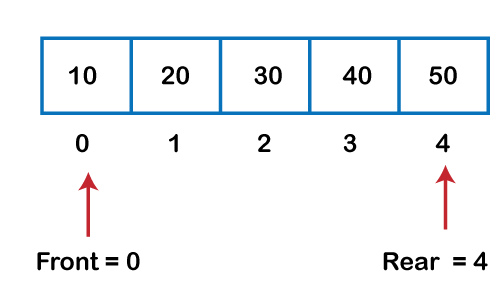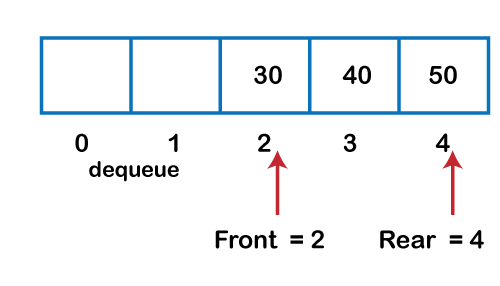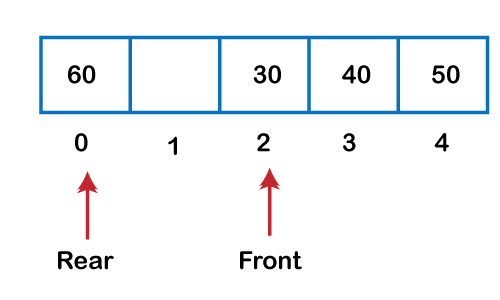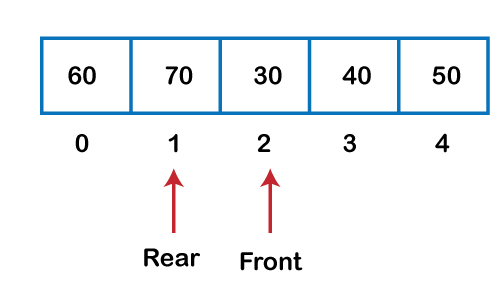### Implementation of circular queue using Array

``````#include <stdio.h>

# define max 6
int queue[max];  // array declaration
int front=-1;
int rear=-1;
// function to insert an element in a circular queue
void enqueue(int element)
{
if(front==-1 && rear==-1)   // condition to check queue is empty
{
front=0;
rear=0;
queue[rear]=element;
}
else if((rear+1)%max==front)  // condition to check queue is full
{
printf("Queue is overflow..");
}
else
{
rear=(rear+1)%max;       // rear is incremented
queue[rear]=element;     // assigning a value to the queue at the rear position.
}
}

// function to delete the element from the queue
int dequeue()
{
if((front==-1) && (rear==-1))  // condition to check queue is empty
{
printf("\nQueue is underflow..");
}
else if(front==rear)
{
printf("\nThe dequeued element is %d", queue[front]);
front=-1;
rear=-1;
}
else
{
printf("\nThe dequeued element is %d", queue[front]);
front=(front+1)%max;
}
}
// function to display the elements of a queue
void display()
{
int i=front;
if(front==-1 && rear==-1)
{
printf("\n Queue is empty..");
}
else
{
printf("\nElements in a Queue are :");
while(i<=rear)
{
printf("%d,", queue[i]);
i=(i+1)%max;
}
}
}
int main()
{
int choice=1,x;   // variables declaration

while(choice<4 && choice!=0)   // while loop
{
printf("\n Press 1: Insert an element");
printf("\nPress 2: Delete an element");
printf("\nPress 3: Display the element");
scanf("%d", &choice);

switch(choice)
{

case 1:

printf("Enter the element which is to be inserted");
scanf("%d", &x);
enqueue(x);
break;
case 2:
dequeue();
break;
case 3:
display();

}}
return 0;
}
``````

Output:

### Implementation of circular queue using linked list

As we know that linked list is a linear data structure that stores two parts, i.e., data part and the address part where address part contains the address of the next node. Here, linked list is used to implement the circular queue; therefore, the linked list follows the properties of the Queue. When we are implementing the circular queue using linked list then both the enqueue and dequeue operations take O(1) time.

``````#include <stdio.h>
// Declaration of struct type node
struct node
{
int data;
struct node *next;
};
struct node *front=-1;
struct node *rear=-1;
// function to insert the element in the Queue
void enqueue(int x)
{
struct node *newnode;  // declaration of pointer of struct node type.
newnode=(struct node *)malloc(sizeof(struct node));  // allocating the memory to the newnode
newnode->data=x;
newnode->next=0;
if(rear==-1)  // checking whether the Queue is empty or not.
{
front=rear=newnode;
rear->next=front;
}
else
{
rear->next=newnode;
rear=newnode;
rear->next=front;
}
}

// function to delete the element from the queue
void dequeue()
{
struct node *temp;   // declaration of pointer of node type
temp=front;
if((front==-1)&&(rear==-1))  // checking whether the queue is empty or not
{
printf("\nQueue is empty");
}
else if(front==rear)  // checking whether the single element is left in the queue
{
front=rear=-1;
free(temp);
}
else
{
front=front->next;
rear->next=front;
free(temp);
}
}

// function to get the front of the queue
int peek()
{
if((front==-1) &&(rear==-1))
{
printf("\nQueue is empty");
}
else
{
printf("\nThe front element is %d", front->data);
}
}

// function to display all the elements of the queue
void display()
{
struct node *temp;
temp=front;
printf("\n The elements in a Queue are : ");
if((front==-1) && (rear==-1))
{
printf("Queue is empty");
}

else
{
while(temp->next!=front)
{
printf("%d,", temp->data);
temp=temp->next;
}
printf("%d", temp->data);
}
}

void main()
{
enqueue(34);
enqueue(10);
enqueue(23);
display();
dequeue();
peek();
}
``````

Output: